Thermodynamics and Propulsion

# 8.5 Rankine Power Cycles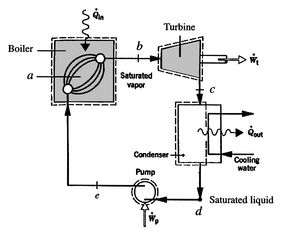A schematic of the components of a Rankine cycle is shown in Figure 8.11. The cycle is shown on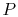-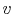,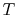-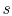, and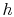-coordinates in Figure 8.12. The processes in the Rankine cycle are as follows:
1.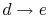: Cold liquid at initial temperatureis pressurized reversibly to a high pressure by a pump. In this process, the volume changes slightly.
2.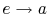: Reversible constant pressure heating in a boiler to temperature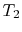.
3.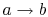: Heat added at constant temperature(constant pressure), with transition of liquid to vapor.
4.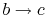: Isentropic expansion through a turbine. The quality decreases from unity at point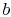to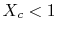.
5.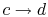: Liquid-vapor mixture condensed at temperatureby extracting heat.

 [-coordinates]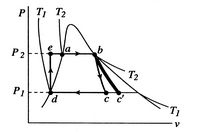[-coordinates]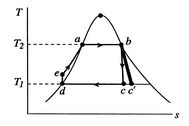[-coordinates]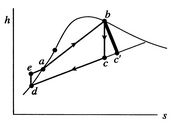In the Rankine cycle, the mean temperature at which heat is supplied is less than the maximum temperature,, so that the efficiency is less than that of a Carnot cycle working between the same maximum and minimum temperatures. The heat absorption takes place at constant pressure over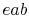, but only the partis isothermal. The heat rejected occurs over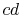; this is at both constant temperature and pressure.

To examine the efficiency of the Rankine cycle, we define a mean effective temperature,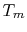, in terms of the heat exchanged and the entropy differences: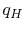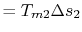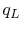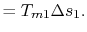The thermal efficiency of the cycle is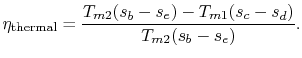The compression and expansion processes are isentropic, so the entropy differences are related by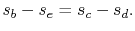The thermal efficiency can be written in terms of the mean effective temperatures as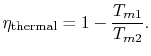For the Rankine cycle,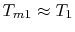,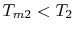. From this equation we see not only the reason that the cycle efficiency is less than that of a Carnot cycle, but the direction to move in terms of cycle design (increased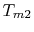) if we wish to increase the efficiency.

There are several features that should be noted about Figure 8.12 and the Rankine cycle in general:

1. The-and the-diagrams are not similar in shape, as they were with the perfect gas with constant specific heats. The slope of a constant pressure reversible heat addition line is, as derived in Chapter 6,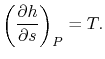In the two-phase region, constant pressure means also constant temperature, so the slope of the constant pressure heat addition line is constant and the line is straight.
2. The effect of irreversibilities is represented by the dashed line fromto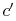. Irreversible behavior during the expansion results in a value of entropy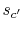at the end state of theexpansion that is higher than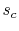. The enthalpy at the end of the expansion (the turbine exit) is thus higher for the irreversible process than for the reversible process, and, as seen for the Brayton cycle, the turbine work is thus lower in the irreversible case.
3. The Rankine cycle is less efficient than the Carnot cycle for given maximum and minimum temperatures, but, as said earlier, it is more effective as a practical power production device.

Muddy Points

Where does degrees Rankine come from? Related to Rankine cycles? (MP 8.9)

UnifiedTP